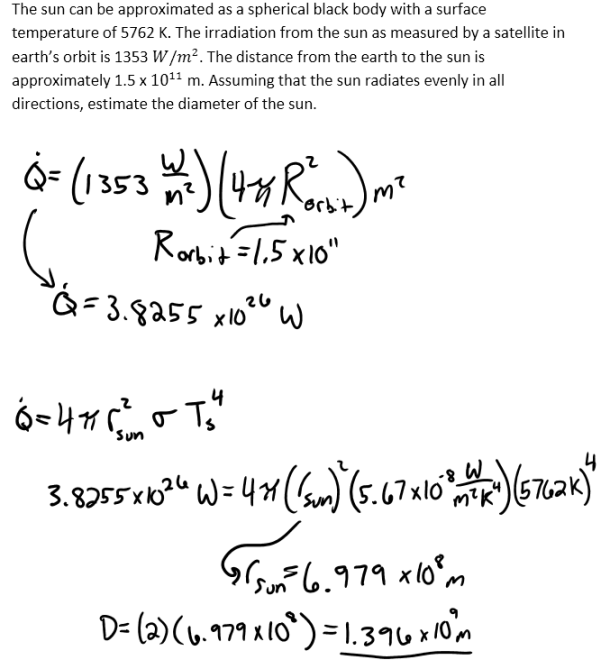heat and mass transfer problems engineering equations heat and mass transfer school homework engineering heat and mass transfer formulas heat and mass transfer solutions to heat and mass transfer problems full solution engineering problem solution heat and mass transfer math problems engineering equations heat and mass transfer school homework engineering solutions to heat and mass transfer formulas heat problems mass problem solutions to transfer problems full solution heat and mass transfer
heat and mass transfer problems engineering equations heat and mass transfer school homework engineering heat and mass transfer formulas heat and mass transfer solutions to heat and mass transfer problems full solution engineering problem solution heat and mass transfer math problems engineering equations heat and mass transfer school homework engineering solutions to heat and mass transfer formulas heat problems mass problem solutions to transfer problems full solution heat and mass transfer
Highalphabet Home Page heat and mass transfer problem solutions Heat and Mass Transfer Page
The sun can be approximated as a spherical black body with a surface temperature of 5762 K. The irradiation from the sun as measured by a satellite in earth’s orbit is 1353 W/m^2. The distance from the earth to the sun is approximately 1.5 x 10^11 m. Assuming that the sun radiates evenly in all directions, estimate the diameter of the sun.The sun can be approximated as a spherical black body with a surface temperature of 5762 K. The irradiation from the sun as measured by a satellite in earth’s orbit is 1353 W/m^2. The distance from the earth to the sun is approximately 1.5 x 10^11 m. Assuming that the sun radiates evenly in all directions, estimate the diameter of the sun.